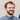# A Quick Primer on Signed vs. Unsigned Integers

Nick Scialli April 19, 2021🚀 4 minute read

If you use high-level programming languages, you may have come across signed and unsigned integers. Here’s a quick primer on what they are.

# Integers Stored as Bits

Under the hood, integers are stored as bits (binary digits), meaning they are represented by a combination of `0` and `1`. If you have a 4-bit integer, there are four slots for 0s and 1s, if you have a 16-bit integer, 16 slots for 0s and 1s.

Here are a few valid 4-bit integers:

• 0000
• 0101
• 1000
• 1110

Every permutation of 0s and 1s will represent a different integer.

# Signedness

So what do these bit combinations represent? Well that depends on whether the integer is signed or unsigned. A signed integer means the number can be negative, zero, or positive and an unsigned integer means the number can only be zero or positive. If your integer can have a negative sign, it’s signed!

Let’s look at how this might work for our 4-bit cases.

## 4-Bit Integer, Signed vs. Unsigned

Encoding Unsigned Signed
0000 0 0
0001 1 1
0010 2 2
0011 3 3
0100 4 4
0101 5 5
0110 6 6
0111 7 7
1000 8 -8
1001 9 -7
1010 10 -6
1011 11 -5
1100 12 -4
1101 13 -3
1110 14 -2
1111 15 -1

Note how our unsigned, 4-bit integer can have a range of 0 to 15 whereas our signed, 4-bit integer can have a range from -8 to 7. In this example, the first bit of the signed integer is known as the sign bit because it determines whether the associated integer is positive or negative—if the first bit is `1`, then the integer is negative.

Indeed, one consideration when determining whether to use a signed or unsigned integer is whether you need to accommodate negative numbers—only signed integers can do this! Of course, you can see that by allowing some of the combinations to represent negative numbers, we have a lower maximum possible value for our integer. A tradeoff!

Note: The way unsigned integers are represented in the above table is known as Two’s complement, which is used on most modern computing devices. You can learn more about other signed number representations on Wikipedia.

# Some Quick Math on Integer Ranges

As I mentioned before, the 4-bit integer has different possible ranges depending on whether the integer is signed or not. If signed, the range is -8 to 7. If unsigned, the range is 0 to 15.

There are formulas for this! Where `n` is the number of bits, the following holds true:

Signed integers can be between -(2n-1) and 2n-1 - 1

Unsigned integers can be between 0 and 2n - 1

Plugging in 4 for n, we can see it holds up in the case we did by hand:

Signed

Min: -(24-1) = -(23) = -8

Max: 24 - 1 - 1 = 8 - 1 = 7

Unsigned

Min: 0

Max: 24 - 1 = 16 -1 = 15

# Conclusion

I hope you learned a little something about integers today! If you found this interesting, I’d encourage you to keep digging into foundational topics as you hear of them.

If you'd like to support this blog by buying me a coffee I'd really appreciate it!Nick Scialli is a software engineer at the U.S. Digital Service.